What is a Moment?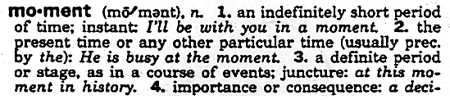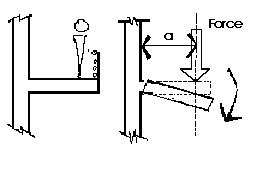The Moment of a force is a measure of its tendency to cause a body to rotate about a specific point or axis. This is different from the tendency for a body to move, or translate, in the direction of the force. In order for a moment to develop, the force must act upon the body in such a manner that the body would begin to twist. This occurs every time a force is applied so that it does not pass through the centroid of the body. A moment is due to a force not having an equal and opposite force directly along it's line of action.

Imagine two people pushing on a door at the doorknob from opposite sides. If both of them are pushing with an equal force then there is a state of equilibrium. If one of them would suddenly jump back from the door, the push of the other person would no longer have any opposition and the door would swing away. The person who was still pushing on the door created a moment.

Elements of a Moment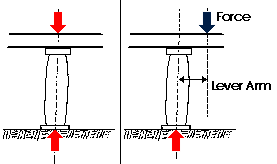The magnitude of the moment of a force acting about a point or axis is directly proportinoal to the distance of the force from the point or axis. It is defined as the product of the force (F) and the moment arm (d). The moment arm or lever arm is the perpendicular distance between the line of action of the force and the center of moments.

Moment = Force x Distance or M = (F)(d)

The Center of Moments may be the actual point about which the force causes rotation. It may also be a reference point or axis about which the force may be considered as causing rotation. It does not matter as long as a specific point is always taken as the reference point. The latter case is much more common situation in structural design problems.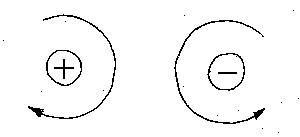A moment is expressed in units of foot-pounds, kip-feet, newton-meters, or kilonewton-meters. A moment also has a sense; A clockwise rotation about the center of moments will be considered a positive moment; while a counter-clockwise rotation about the center of moments will be considered negative. The most common way to express a moment is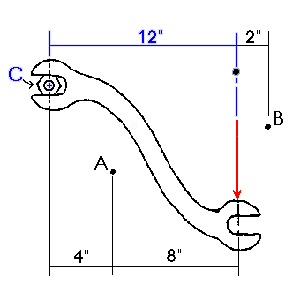The example shows a wrench being applied to a nut. A 100 pound force is applied to it at point C, the center of the nut. The force is applied at an x- distance of 12 inches from the nut. The center of moments could be point C, but could also be points A or B or D.

The moment arm for calculating the moment around point C is 12 inches. The magnitude of the moment about point C is 12 inches multiplied by the force of 100 lbs to give a total moment of 1200 inch-lbs (or 100 ft-lbs).

Moment Arm (d) = 12 inches

Magnitude (F) = 100 lbs

Moment = M = 100 lbs x 12 in. = 1200 in-lbs

Similarly, we can find the moments about any point in space.

 Moment @ A B D Moment Arm 8 inches 2 inches 0 inches Magnitude of F 100 pounds 100 pounds 100 pounds Total Moment 800 in- pounds 200 in- pounds 0 in- pounds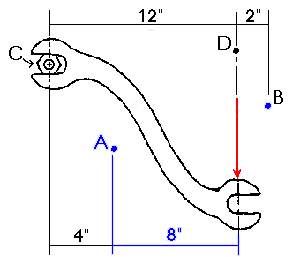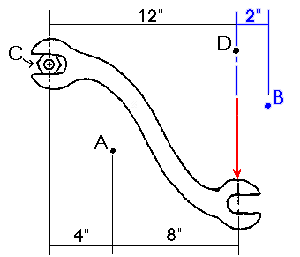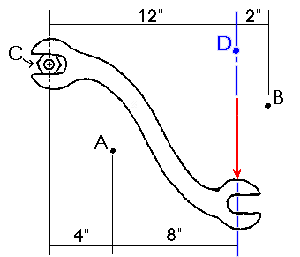A moment causes a rotation about a point or axis. If the moment is to be taken about a point due to a force F, then in order for a moment to develop, the line of action cannot pass through that point. If the line of action does go through that point, the moment is zero because the magnitude of the moment arm is zero. Such was the case for point D in the previous wrench poblem. The total moment was zero because the moment arm was zero as well.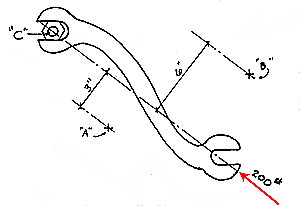As another example, let us assume that 200 pound force is applied to the wrench as indicated. The moment of the 200 pound force applied at C is zero because:

M = F x d = 200 lbs x 0 in = 0 in-lbs

In other words, there is no tendency for the 200 pound force to cause the wrench to rotate the nut. One could increase the magnitude of the force until the bolt finally broke off (shear failure).

The moment about points X, Y, and Z would also be zero because they also lie on the line of action.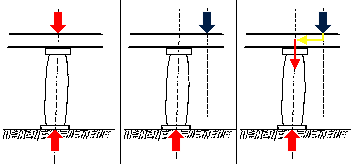A moment can also be considered to be the result of forces detouring from a direct line drawn between the point of loading of a system and its supports. In this case, the blue force is an eccentric force. In order for it to reach the base of the column, it must make a detour through the beam. The greater the detour, the greater the moment. The most efficient structural systems have the least amount of detours possible. This will be discussed in more detail in Lecture 37 and later courses.

There are cases in which it is easier to calculate the moments of the componenets of a force around a certain point than it is to calculate the moment of the force itself. It could be that the determination of the perpendicular distance of the force is more difficult than determining the perpendicular distance of components of the force. The moment of several forces about a point is simply the algebraic sum of their component moments about the same point. When adding the moments of componenets, one must take great care to be consistant with the sense of each moment. It is often prudent to note the sense next to the moment when undertaking such problems.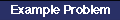Combined MomentsMoments on a Beam

Common Mistakes
When adding the moments of componenets, one must take great care to be consistant with the sense of each moment. It is often prudent to note the sense next to the moment when undertaking such problems.

Any difficulty with calculating a moment can usually be traced to one of the following:

• The center of moments has not been correctly established or clearly understood.
• The assumed moment arm is not the PERPENDICULAR distance between the line of action of the force and the center of moments.
• The direction, or sense, of the rotation has been ignored or misunderstood.

Questions For Thought
What is the moment about point B and about point D for both of the cases shown in the wrench example above? How could adding an extension to the end of the wrench help turn a rusted bolt? What kind of structural systems would have the least number of "detours?"

Problems